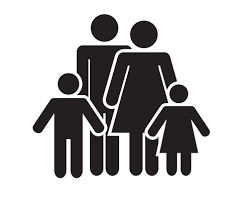Large family

The average age of all family members (children, mother, father, grandmother, grandfather) is 29 years. The average age of parents is 40 years, grandparents 66 years and all children are 5 years. How many children are there in this family?

n =  4

Step-by-step explanation:

29•(n+2+2) = 5n+2•40+2•66

29•(n+2+2) = 5•n+2•40+2•66

24n = 96

n = 4

Our simple equation calculator calculates it.Did you find an error or inaccuracy? Feel free to write us. Thank you!Tips to related online calculators
Looking for help with calculating arithmetic mean?
Looking for a statistical calculator?
Do you have a linear equation or system of equations and looking for its solution? Or do you have a quadratic equation?
Do you want to convert time units like minutes to seconds?

You need to know the following knowledge to solve this word math problem:

We encourage you to watch this tutorial video on this math problem: